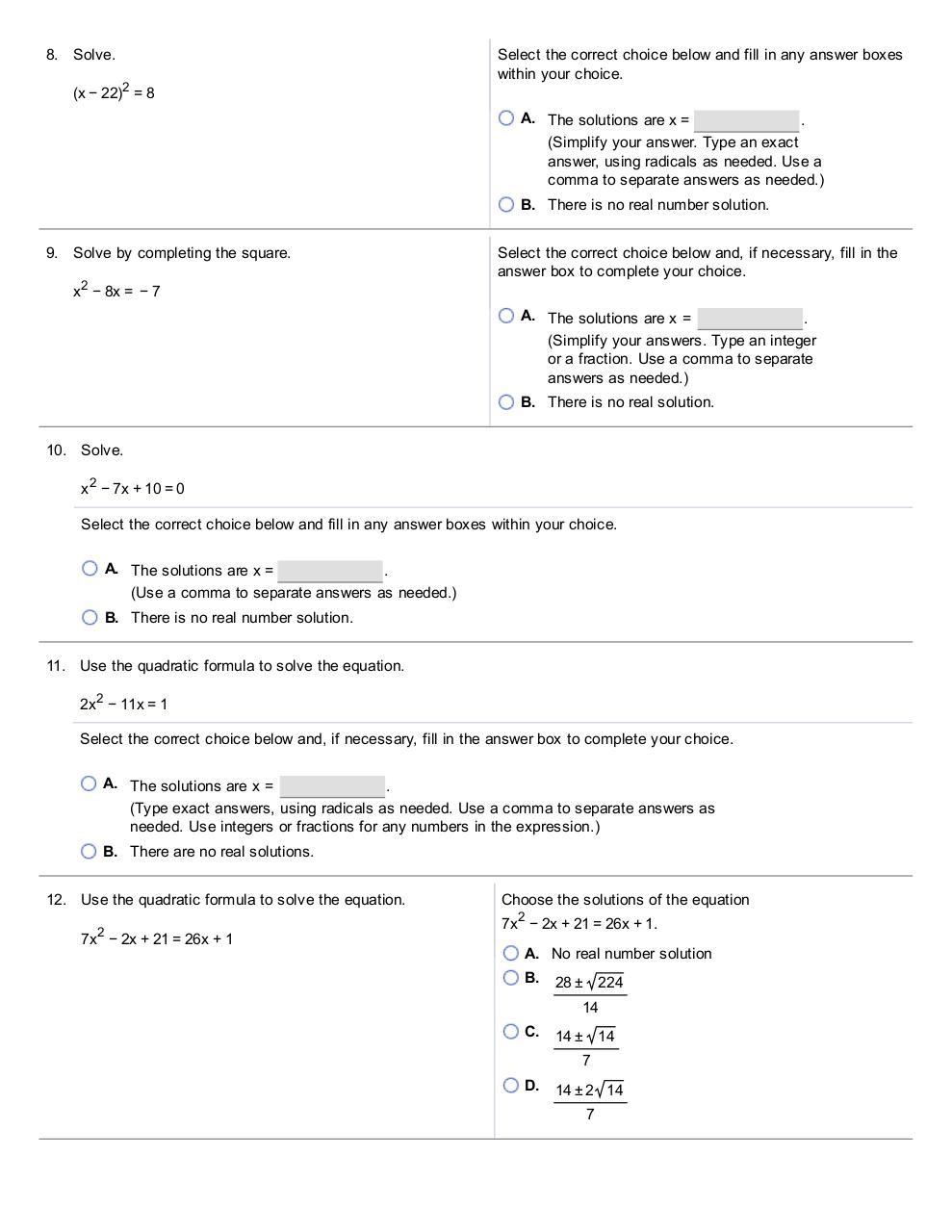# College Level.pdfPage 1 2 3 4 5 6 7 8 9 10

#### Text preview

8. Solve.
(x − 22 )2 = 8

Select the correct choice below and fill in any answer boxes
within your choice.
A. The solutions are x =
.
(Simplify your answer. Type an exact
comma to separate answers as needed.)
B. There is no real number solution.

9. Solve by completing the square.
x 2 − 8x = − 7

Select the correct choice below and, if necessary, fill in the
answer box to complete your choice.
A. The solutions are x =
.
(Simplify your answers. Type an integer
or a fraction. Use a comma to separate
B. There is no real solution.

10. Solve.
x 2 − 7x + 10 = 0
Select the correct choice below and fill in any answer boxes within your choice.
A. The solutions are x =

(Use a comma to separate answers as needed.)
B. There is no real number solution.
11. Use the quadratic formula to solve the equation.
2x 2 − 11x = 1
Select the correct choice below  and, if  necessary, fill in the answer box to complete your choice.
A. The solutions are x  =

needed. Use integers or fractions for any numbers in the  expression.)
B. There are no real solutions.
12. Use the quadratic formula to solve the equation.

Choose the solutions of the equation
2

2

7x − 2x + 21 = 26x + 1

7x − 2x + 21 = 26x + 1.
A. No real number solution
B.

28 ±

224

14
C.

14 ±

14

7
D.

14 ± 2 14
7